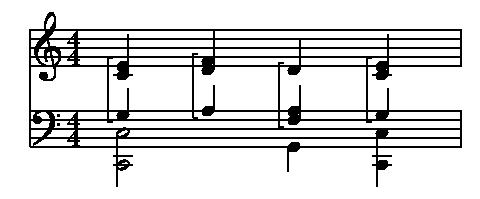## Marking complicated tempo changes

Sometimes you may wish to indicate tempo changes by showing 2 note values with an equals sign between them. For simple cases, this is fairly straightforward, but if you want to include beamed notes or a triplet, a little more work is required. Another use of this notation is to show that the music is to be played in "swing time," as in this example, which has two ordinary eight notes on one side of the equals sign, and a triplet made up of a quarter and eighth note on the other:

```score
// This example assumes "size" is set to 15
size=15
beamstyle=4,4

music

// set a location tag
1: [=c] 8c+;a;g;f;

// set X and Y to where the first note will be placed
define X c.x - 3 @
define Y c.y + 5 @

// print "straight 8ths = triplet quarter plus 8th"

// print the basic notes and equal sign
print (X, Y) "\(smup4n)  \(smup4n)  = \(smup4n)  \(smup8n)"

// print the beam between the straight 8th notes
wide line (X + 2.1, Y + 5.5) to (X + 7.2, Y + 5.5)

// now do the triplet bracket
line (X + 14.7, Y + 7.0)  to (X + 17.6, Y + 7.0) // horz left top segment
line (X + 19.4, Y + 7.0)  to (X + 22.5, Y + 7.0) // horz right top segment
line (X + 14.7, Y + 7.05) to (X + 14.7, Y + 5.3) // vert left side segment
line (X + 22.5, Y + 7.05) to (X + 22.5, Y + 5.3) // vert right side segment

// print the 3 in the middle of the bracket
print (X + 17.9, Y + 6.1) "\s(8)\f(TI)3"	// the 3 for the triplet

bar
```Note that if you want a piece to be played in swing time, you may also want to set the swingunit parameter, which will make the MIDI output use swing time.